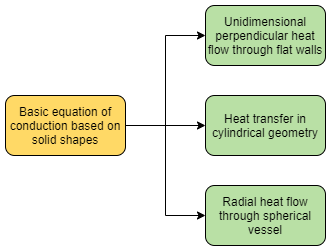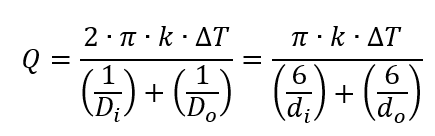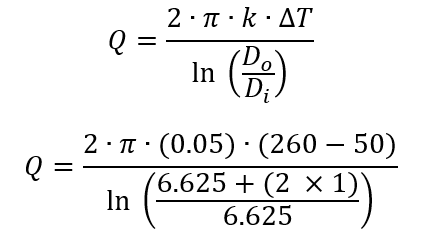# What is Conduction and Its Problem Example

In this post I want to share what is conduction and its problem example.

In contrast to general molecular motion or mixing, conduction describes the rate of heat transfer through materials as a function of vibrations and interactions between nearby molecules. Conduction always applies to solids and rarely to fluids.

There are several fundamental equations for steady heat conduction through some basic solid shapes, neglecting conditions of border:## Conduction in Unidimensional Perpendicular Heat Flow Through Flat Walls

For unidimensional perpendicular heat flow through flat walls, as in heat flow through square or very large cylindrical tank walls, the equation is:Where:

Q        = heat transfer (Btu/hr)

k         = thermal conductivity (Btu/h∙ft∙oF)

A        = area (ft2)

∆T      = temperature difference (oF)

wt       = wall thickness (in)

## Conduction in Cylindrical Geometry

For heat transfer in cylindrical geometry where the heat transfer is normal to the axis, as in heat flow through a cylindrical vessel or pipe wall:Where:

L         = length of heat transfer surface (ft)

Do       = outside diameter of pipe or cylinder (ft)

Di       = inside diameter of pipe or cylinder (ft)

do           = outside diameter of pipe or cylinder or fin (ft)

di        = inside diameter of pipe or cylinder or fin (ft)

## Conduction in Spherical Vessel

For radial heat flow through a spherical vessel:## Example of Conduction Problem

This example shows how to estimate heat loss or heat rate in spherical pipe wall.

Estimate the loss per linear foot through a one inch layer of block insulation covering an 6 in schedule 40 steam header. Assume:

Ti        = 260oF

To       = 50oF

k         = 0.05 Btu/(h∙ft2oF/ft)

Solution

Use equation below to estimate heat transfer:Q = 250.08 Btu/hr per linear ft

That’s all about what is conduction and its problem example. I hope you find this simple post useful.